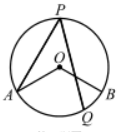$\text{A.}$ $50^{\circ}$ $\text{B.}$ $55^{\circ}$ $\text{C.}$ $60^{\circ}$ $\text{D.}$ $65^{\circ}$

D